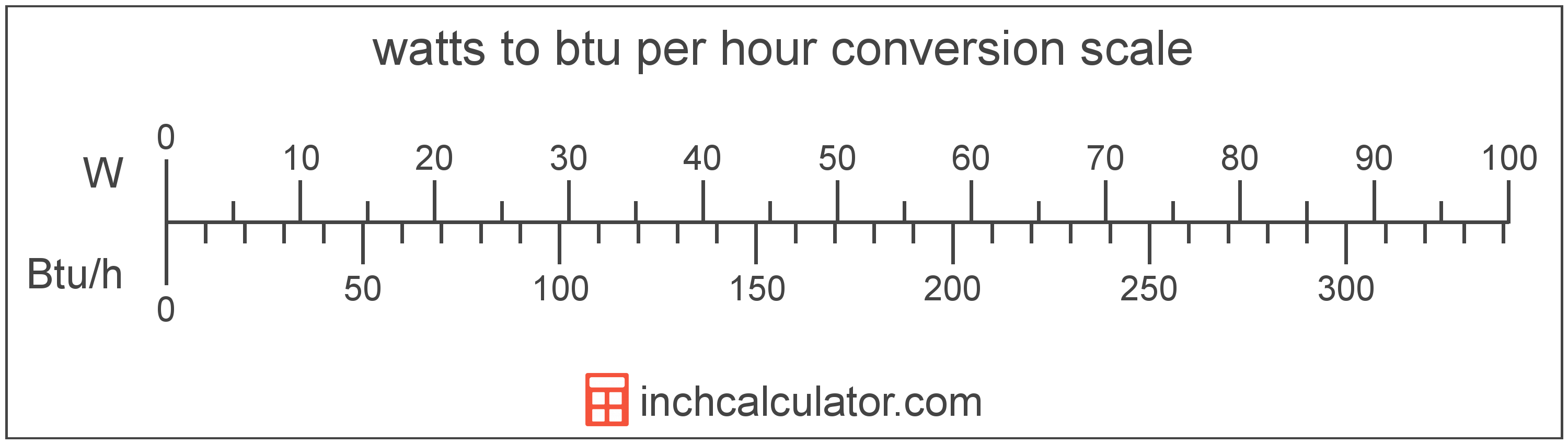# Watts to Btu per Hour Converter

Enter the power in watts below to get the value converted to btu per hour.

Results in Btu per Hour:1,000 W = 3,412.14245 Btu/h

Do you want to convert btu per hour to watts?

## How to Convert Watts to Btu per Hour

To convert a measurement in watts to a measurement in btu per hour, multiply the power by the following conversion ratio: 3.412142 btu per hour/watt.

Since one watt is equal to 3.412142 btu per hour, you can use this simple formula to convert:

btu per hour = watts × 3.412142

The power in btu per hour is equal to the power in watts multiplied by 3.412142.

For example, here's how to convert 5 watts to btu per hour using the formula above.
btu per hour = (5 W × 3.412142) = 17.060712 Btu/h### How Many Btu per Hour Are in a Watt?

There are 3.412142 btu per hour in a watt, which is why we use this value in the formula above.

1 W = 3.412142 Btu/h

## What Is a Watt?

One watt is the power equal to the rate of energy of one joule per second in an electrical circuit. One watt is also equal to the power produced in a circuit with a voltage potential of one volt with a current of one amp.

The watt is the SI derived unit for power in the metric system. Watts can be abbreviated as W; for example, 1 watt can be written as 1 W.

Watt's Law states the relationship between power, current, and voltage. Using Watt's Law, it's possible to express the power in watts as an expression using current and voltage.

PW = IA × VV

The power in watts is equal to the current in amperes times the potential difference in volts.

Combining this with Ohm's Law, it's also possible to express the power in watts using resistance in addition to volts and amps.

## What Are Btu per Hour?

One BTU per hour is equal to one british thermal unit, or the energy needed to heat one pound of water 1 °F, for one hour.

The btu per hour is a US customary unit of power. Btu per hour can be abbreviated as Btu/h; for example, 1 btu per hour can be written as 1 Btu/h.

In the expressions of units, the slash, or solidus (/), is used to express a change in one or more units relative to a change in one or more other units.

## Watt to Btu per Hour Conversion Table

Table showing various watt measurements converted to btu per hour.
Watts Btu Per Hour
1 W 3.4121 Btu/h
2 W 6.8243 Btu/h
3 W 10.24 Btu/h
4 W 13.65 Btu/h
5 W 17.06 Btu/h
6 W 20.47 Btu/h
7 W 23.88 Btu/h
8 W 27.3 Btu/h
9 W 30.71 Btu/h
10 W 34.12 Btu/h
11 W 37.53 Btu/h
12 W 40.95 Btu/h
13 W 44.36 Btu/h
14 W 47.77 Btu/h
15 W 51.18 Btu/h
16 W 54.59 Btu/h
17 W 58.01 Btu/h
18 W 61.42 Btu/h
19 W 64.83 Btu/h
20 W 68.24 Btu/h
21 W 71.65 Btu/h
22 W 75.07 Btu/h
23 W 78.48 Btu/h
24 W 81.89 Btu/h
25 W 85.3 Btu/h
26 W 88.72 Btu/h
27 W 92.13 Btu/h
28 W 95.54 Btu/h
29 W 98.95 Btu/h
30 W 102.36 Btu/h
31 W 105.78 Btu/h
32 W 109.19 Btu/h
33 W 112.6 Btu/h
34 W 116.01 Btu/h
35 W 119.42 Btu/h
36 W 122.84 Btu/h
37 W 126.25 Btu/h
38 W 129.66 Btu/h
39 W 133.07 Btu/h
40 W 136.49 Btu/h

## References

1. International Bureau of Weights and Measures, The International System of Units, 9th Edition, 2019, https://www.bipm.org/documents/20126/41483022/SI-Brochure-9-EN.pdf